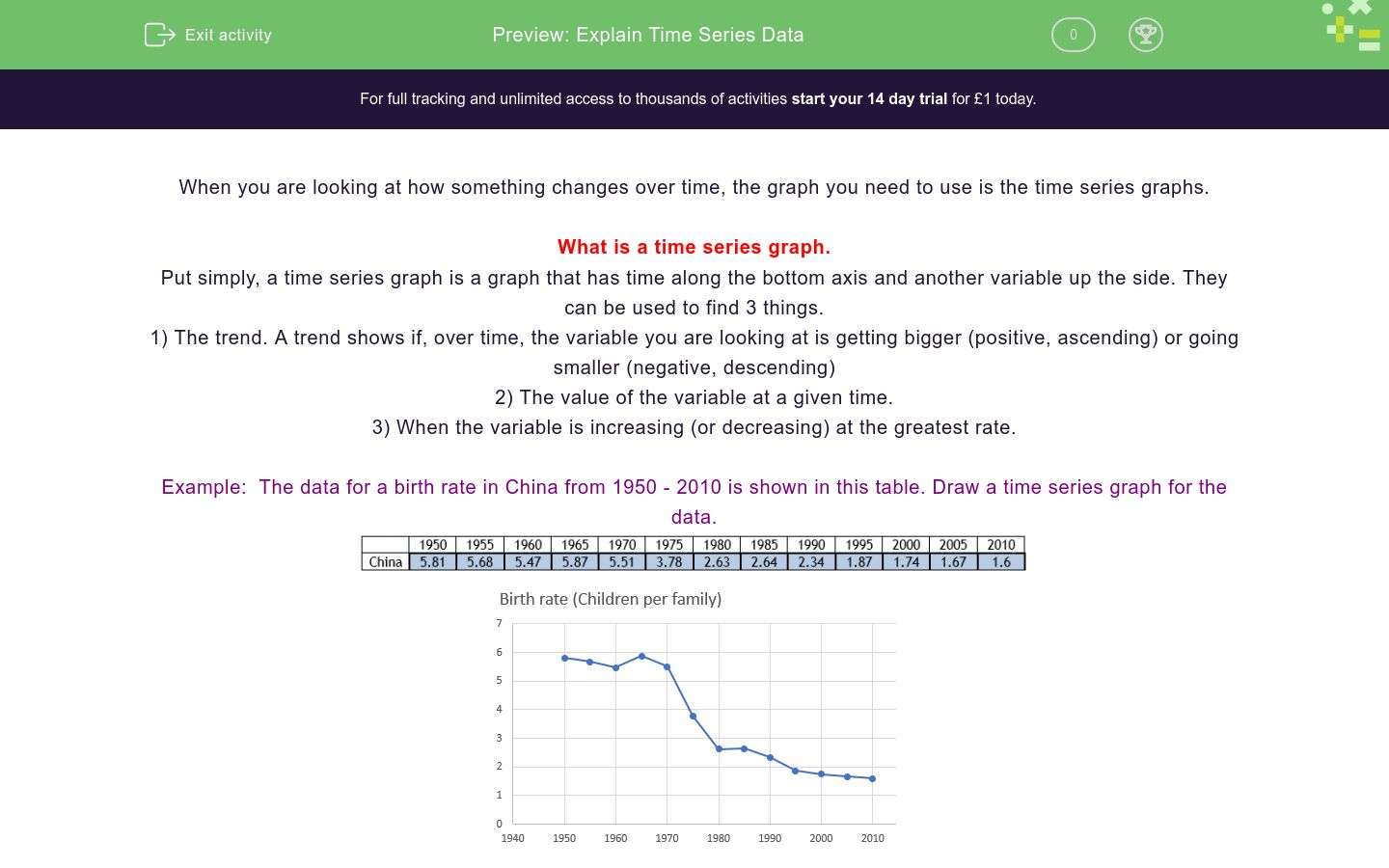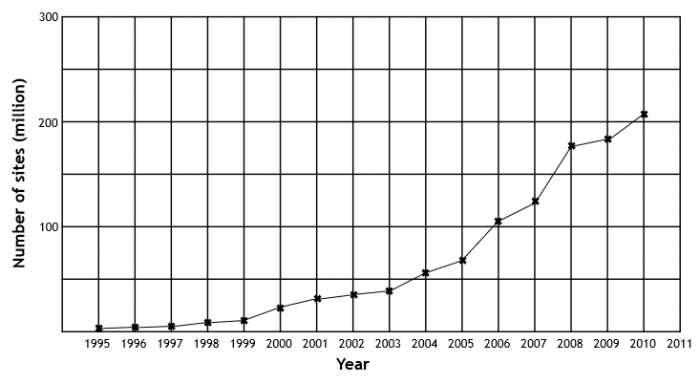# Explain Time Series Data

In this worksheet, pupils practice reading data from time-series graphs.Key stage:  KS 4

GCSE Subjects:   Maths

GCSE Boards:   AQA, Eduqas, Pearson Edexcel, OCR

Curriculum topic:   Statistics

Curriculum subtopic:   Statistics, Interpreting and Representing Data

Difficulty level:### QUESTION 1 of 10

When you are looking at how something changes over time, the graph you need to use is the time series graphs.

What is a time series graph.

Put simply, a time series graph is a graph that has time along the bottom axis and another variable up the side. They can be used to find 3 things.

1) The trend. A trend shows if, over time, the variable you are looking at is getting bigger (positive, ascending) or going smaller (negative, descending)

2) The value of the variable at a given time.

3) When the variable is increasing (or decreasing) at the greatest rate.

Example:  The data for a birth rate in China from 1950 - 2010 is shown in this table. Draw a time series graph for the data.a) Describe the trend for the birth rate in China over time.

There is only one of two words you need here, increasing or decreasing. As the trend for the graph is downwards, we describe this as a decreasing trend.

b) Estimate the birth rate in 1972.

If we read up from 1972 on the graph and then read across, we get the answer of 5 children per family.

c) Between which two years did the birth rate decrease the fastest?

This is actually easier than it sounds. All we need to do is to look for where the line is the steepest.

This would be between 1970 and 1975

A time series graph can be used to find if a trend is...

The following time-series graph shows the number of websites from the year 1995 to 2010To the nearest million, how many websites were there in 2006?

The following time-series graph shows the child mortality rate in Africa from 1995 to 2010Is the trend for child mortality rate over time...

Increasing

Decreasing

The following time-series graph shows the number of websites from the year 1995 to 2010Between which two years did the number of websites increase at the highest rate?

1995

1996

1997

1998

1999

2000

2001

2002

2003

2004

2005

2006

2007

2008

2009

2010

The following time-series graph shows the child mortality rate in Africa from 1995 to 2010What was the child mortality rate in 1970.

The following time-series graph shows the number of websites from the year 1995 to 2010Is the trend for the number of websites over time...

Increasing

Decreasing

The following time-series graph shows the child mortality rate in Africa from 1995 to 2010Between which two years did the number of websites increase at the highest rate?

1955

1960

1965

1970

1975

1980

1985

1990

1995

2000

2005

2010

The following time-series graph shows the percentage of crimes solved in the UK from 2003 to 2010What was the percentage of crimes solved in 2004

The following time-series graph shows the percentage of crimes solved in the UK from 2003 to 2010Is the trend for the percentage of crimes that are solved over time...

Increasing

Decreasing

The following time-series graph shows the percentage of crimes solved in the UK from 2003 to 2010Between which two years did the percentage of crimes that are solved increase at the highest rate?

2003

2004

2005

2006

2007

2008

2009

2010

• Question 1

A time series graph can be used to find if a trend is...

EDDIE SAYS
The general pattern (also called the trend) is either going up or down, we call these increasing and decreasing trends.
• Question 2

The following time-series graph shows the number of websites from the year 1995 to 2010To the nearest million, how many websites were there in 2006?

101
102
103
104
105
106
107
108
109
EDDIE SAYS
If we read up from the year 2006 and across, we get a number just over 100 million. Remember that you will get a margin of error for this as you are reading from a graph.
• Question 3

The following time-series graph shows the child mortality rate in Africa from 1995 to 2010Is the trend for child mortality rate over time...

Decreasing
EDDIE SAYS
If it's going down, its...
• Question 4

The following time-series graph shows the number of websites from the year 1995 to 2010Between which two years did the number of websites increase at the highest rate?

2007
2008
EDDIE SAYS
When we're looking for the increase to be as large as possible, we just need to say between which two years the lines is steepest.
• Question 5

The following time-series graph shows the child mortality rate in Africa from 1995 to 2010What was the child mortality rate in 1970.

227
228
229
230
231
232
233
EDDIE SAYS
If we read up from the year 1970 and across, we get a number around about 230 Remember that you will get a margin of error for this as you are reading from a graph.
• Question 6

The following time-series graph shows the number of websites from the year 1995 to 2010Is the trend for the number of websites over time...

Increasing
EDDIE SAYS
If it's going up, its...
• Question 7

The following time-series graph shows the child mortality rate in Africa from 1995 to 2010Between which two years did the number of websites increase at the highest rate?

1975
1980
EDDIE SAYS
When we're looking for the decrease to be as large as possible, we just need to say between which two years the lines is steepest.
• Question 8

The following time-series graph shows the percentage of crimes solved in the UK from 2003 to 2010What was the percentage of crimes solved in 2004

25
25%
25 %
EDDIE SAYS
If we read up from the year 2004 and across, we get exactly 25% Most of the time when you are reading from a graph, you will get a margin of error. On this one, because you hit an exact value, you don't get a margin, your number has to be exact.
• Question 9

The following time-series graph shows the percentage of crimes solved in the UK from 2003 to 2010Is the trend for the percentage of crimes that are solved over time...

Increasing
EDDIE SAYS
If it's going up, its...
• Question 10

The following time-series graph shows the percentage of crimes solved in the UK from 2003 to 2010Between which two years did the percentage of crimes that are solved increase at the highest rate?

2008
2009
EDDIE SAYS
When we're looking for the decrease to be as large as possible, we just need to say between which two years the lines is steepest.
---- OR ----

Sign up for a £1 trial so you can track and measure your child's progress on this activity.

### What is EdPlace?

We're your National Curriculum aligned online education content provider helping each child succeed in English, maths and science from year 1 to GCSE. With an EdPlace account you’ll be able to track and measure progress, helping each child achieve their best. We build confidence and attainment by personalising each child’s learning at a level that suits them.

Get started